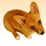# export to jpeg loop

2702
3
Jump to solution
03-30-2012 05:23 AMNew Contributor
I created a loop to zoom to every shape in a shapefile and export that particular extent. The only problem is that the jpeg is given the number of the row as name. I want to give it the name of a field in de dbf. Does anybody have an idea how to do that?

import arcpy
from arcpy import env
from arcpy import mapping

mxd = arcpy.mapping.MapDocument(r"C:\gisbestanden\pythontest\test13.mxd")
df = arcpy.mapping.ListDataFrames(mxd)

fc = r"Z:\kaarten\COROP\coropmultipart.shp"
count = str(arcpy.GetCount_management(fc))

x = 0

while x < int(count) + 1:
rows = arcpy.SearchCursor(fc, "fid = " + str(x))
for row in rows:
xmin = row.shape.extent.XMin
ymin = row.shape.extent.YMin
xmax = row.shape.extent.XMax
ymax = row.shape.extent.YMax
df = arcpy.mapping.ListDataFrames(mxd)
newExtent = df.extent
newExtent.XMin, newExtent.YMin = xmin, ymin
newExtent.XMax, newExtent.YMax = xmax, ymax
df.extent = newExtent
mapping.ExportToJPEG(mxd, r"C:\gisbestanden\pythontest\gemeentekaart\JPEG_" + str(x) + ".jpg")

print "successfully printed JPG"
x += 1
Tags (2)
1 Solution

Accepted SolutionsbyMVP Esteemed Contributor
I created a loop to zoom to every shape in a shapefile and export that particular extent. The only problem is that the jpeg is given the number of the row as name. I want to give it the name of a field in de dbf. Does anybody have an idea how to do that?

This is done the same way you accessed the shape field:

`import arcpyfrom arcpy import envfrom arcpy import mappingmxd = arcpy.mapping.MapDocument(r"C:\gisbestanden\pythontest\test13.mxd")df = arcpy.mapping.ListDataFrames(mxd)fc = r"Z:\kaarten\COROP\coropmultipart.shp"  count = str(arcpy.GetCount_management(fc))x = 0while x < int(count) + 1:    rows = arcpy.SearchCursor(fc, "fid = " + str(x))     for row in rows:        xmin = row.shape.extent.XMin        ymin = row.shape.extent.YMin        xmax = row.shape.extent.XMax        ymax = row.shape.extent.YMax        # get the field contents to name the file        filename = row.getValue("NAMEFIELD") # or: row.NAMEFIELD        df = arcpy.mapping.ListDataFrames(mxd)        newExtent = df.extent        newExtent.XMin, newExtent.YMin = xmin, ymin        newExtent.XMax, newExtent.YMax = xmax, ymax        df.extent = newExtent        # use the field contents in the filename        mapping.ExportToJPEG(mxd,              r"C:\gisbestanden\pythontest\gemeentekaart\JPEG_{0}.jpg".format(filename))        print "successfully printed JPG"    x += 1`
3 RepliesbyMVP Esteemed Contributor
I created a loop to zoom to every shape in a shapefile and export that particular extent. The only problem is that the jpeg is given the number of the row as name. I want to give it the name of a field in de dbf. Does anybody have an idea how to do that?

This is done the same way you accessed the shape field:

`import arcpyfrom arcpy import envfrom arcpy import mappingmxd = arcpy.mapping.MapDocument(r"C:\gisbestanden\pythontest\test13.mxd")df = arcpy.mapping.ListDataFrames(mxd)fc = r"Z:\kaarten\COROP\coropmultipart.shp"  count = str(arcpy.GetCount_management(fc))x = 0while x < int(count) + 1:    rows = arcpy.SearchCursor(fc, "fid = " + str(x))     for row in rows:        xmin = row.shape.extent.XMin        ymin = row.shape.extent.YMin        xmax = row.shape.extent.XMax        ymax = row.shape.extent.YMax        # get the field contents to name the file        filename = row.getValue("NAMEFIELD") # or: row.NAMEFIELD        df = arcpy.mapping.ListDataFrames(mxd)        newExtent = df.extent        newExtent.XMin, newExtent.YMin = xmin, ymin        newExtent.XMax, newExtent.YMax = xmax, ymax        df.extent = newExtent        # use the field contents in the filename        mapping.ExportToJPEG(mxd,              r"C:\gisbestanden\pythontest\gemeentekaart\JPEG_{0}.jpg".format(filename))        print "successfully printed JPG"    x += 1`New Contributor

Hi Curtis,

I'm new to Python, but this is exactly what I need to do - run through my shapefile (poly) and zoom to each polygon within and export to JPEG. I've copied your script, but keep getting the attribute error 'Extent' object has no attribute 'Xmin'. My shapefile consists of 4 fields (FID, Shape, Unit, Hectares), am I missing something?

NateNew Contributor
thanks for the quick reply! It worked!!